# Random Pythagoras trees

Just for Godfried: a forest of random Pythagoras trees. Here is my Postscript code for one of the trees.

I took each random vertex uniformly on a halfcircle, but one could consider other distributions as well. Here are some theoretical questions related to it.

• Consider n generations of squares (2^n squares in the last level considered), choose one of these squares uniformly at random, and let X_n denote its location. What is the limit distribution of X_n as n tends to infinity? (Easy.)
• If I let each branch grow until its last square reaches area 1/n, then what is the path length of the longest branch (as a function of n)? (Hint: can you find a random binary search tree in there?)
• What is the limit law of the maximal distance from a square in generation n to the origin? (Hard.)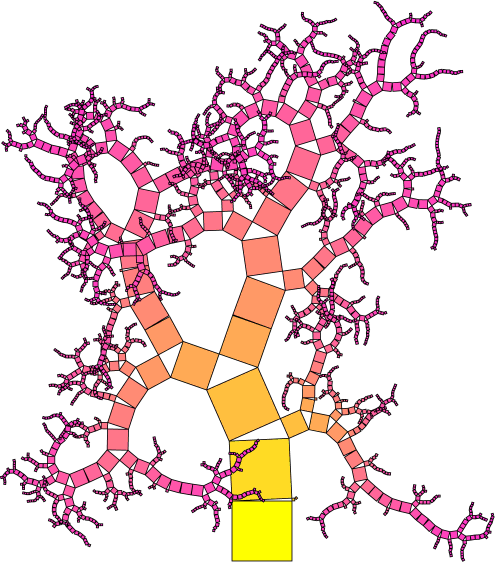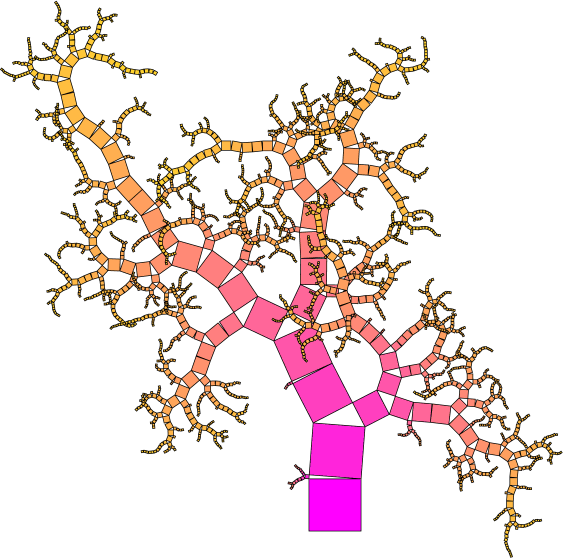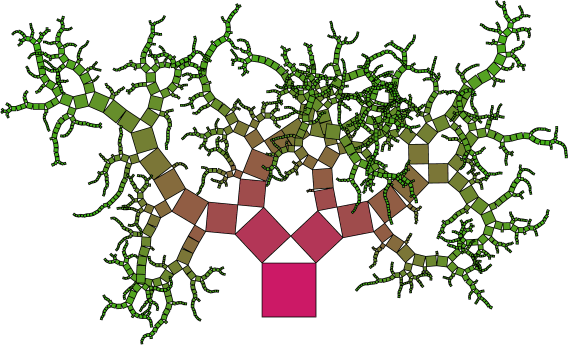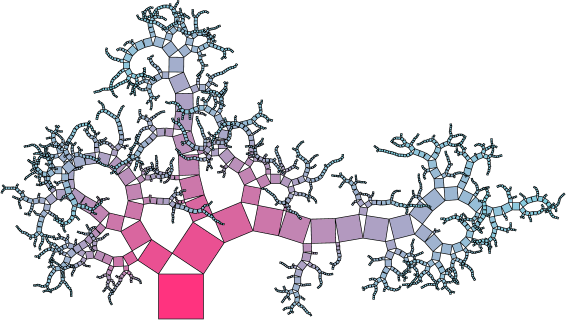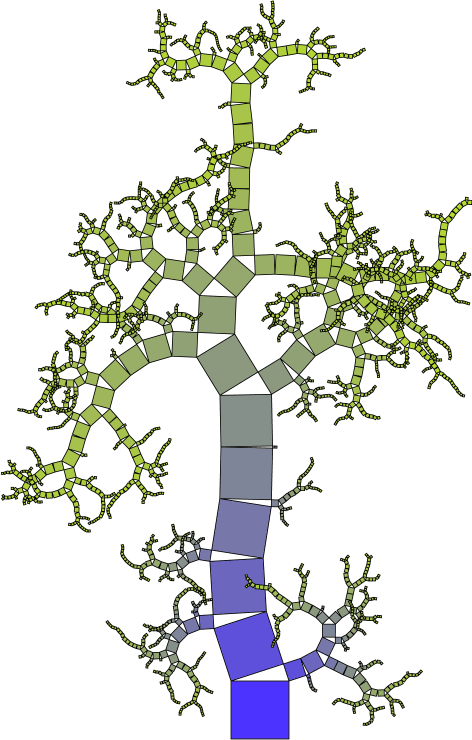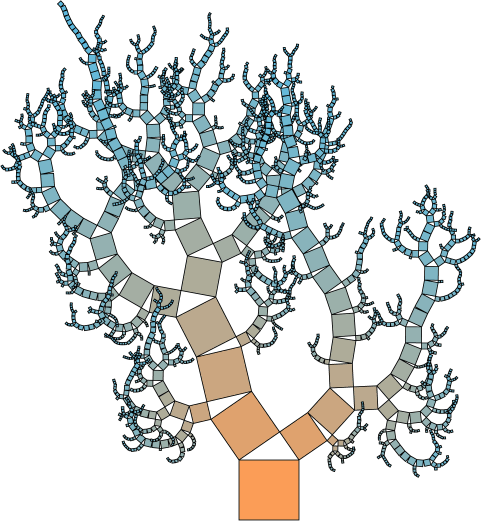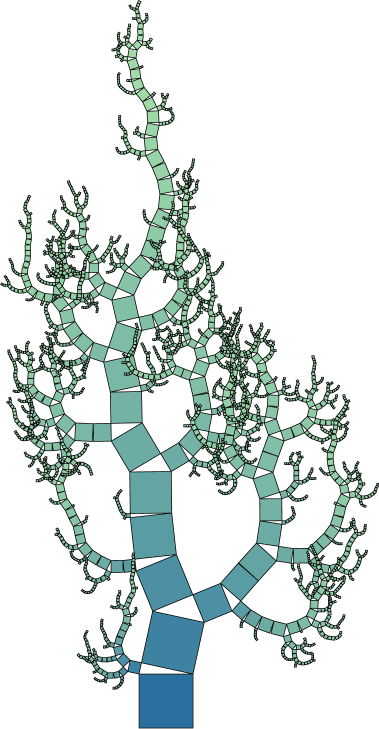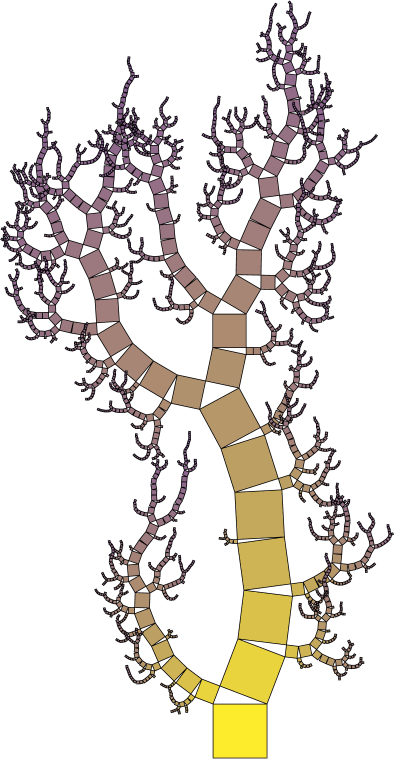In the last three trees, I flipped triangles to make the largest branch always grow most vertically up.

 Contact Luc Devroye School of Computer Science McGill University Montreal, Canada H3A 2K6 luc@cs.mcgill.ca http://cg.scs.carleton.ca/~luc